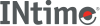﻿ pdt (SDM)INtime SDK Help
pdt (SDM)

Displays one or more entries from a page table, which points to memory pages holding a flat-model application's code and data. for the meaning of each entry in the display, see the description of the `.component` parameter.

Paging information in the user's manual or programmer's reference for your microprocessor.

```[count] pdt [(index) [.component]] physical_base
```

#### Parameters

`count`
A decimal number specifying how many entries from the page table to display. If you don't specify `count` or `(index)`, the default is to display a screenful of entries until you type `Q` to quit. You can increment the display one screen at a time by typing <Space>, or one line at a time by typing <CR>. If you don't specify `count` but do specify `(index)`, the default value for count is 1.
`(index)`
The index into the table to begin the display. For example, assume that the page table's base address is 25A000H. To display 5 entries beginning with entry 10, you would enter:
```5pdt(10t) 25a000
```
`.component`
To display only a single component of a specific directory entry, after the `(index)` enter a period (.) followed by one of these component abbreviations:
 `ADDR` Base address of the page. `AV` Available bits for use by the OS. `D` Dirty bit: 1 means memory in the page has been written. `A` Accessed bit: 1 means read or write to the page. `PCD` Page-level Cache Disable bit: 1 means caching is disabled. `PWT` Page-level WriteThrough bit: 1 is writethrough, 0 is writeback. `U` User/Supervisor bit: 1 is application code and data, 0 is OS memory. `W` Read/Write bit: 1 is read/write, 0 is read-only. `P` Present bit: 1 means the page is present in memory.
`physical_base`
The base address of the page table, as displayed by the pdd command.

#### Examples

1. Assume that a pdd command displayed the base address of a page table as 00276000. To get information about the memory pages in that page table, use the following command:
```..pdt 276000
PTBL(0T) ADDR=00c00000 AV=0 D=0 A=0 PCD=0 PWT=0 U=1 W=1 P=1
PTBL(1T) ADDR=00c01000 AV=0 D=0 A=0 PCD=0 PWT=0 U=1 W=1 P=1
PTBL(2T) ADDR=00c02000 AV=0 D=0 A=0 PCD=0 PWT=0 U=1 W=1 P=1
PTBL(3T) ADDR=00c03000 AV=0 D=0 A=0 PCD=0 PWT=0 U=1 W=1 P=1
PTBL(4T) ADDR=00c04000 AV=0 D=0 A=0 PCD=0 PWT=0 U=1 W=1 P=1
PTBL(5T) ADDR=00c05000 AV=0 D=0 A=0 PCD=0 PWT=0 U=1 W=1 P=1
.
.
.
PTBL(21T) ADDR=00c15000 AV=0 D=0 A=0 PCD=0 PWT=0 U=1 W=1 P=1
Enter <Space> or <CR> or Quit -
```
2. To get just the base address of the 6th page (index 5) in that page table, use the following command.
```..pdt(5t).addr 276000